# First man

What is the likelihood of a random event where are five men and seven women first will leave the man?

Correct result:

p =  0.4167

#### Solution:

$p=5\mathrm{/}\left(5+7\right)=\frac{5}{12}=0.4167$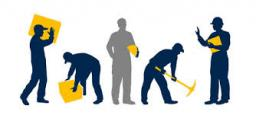We would be pleased if you find an error in the word problem, spelling mistakes, or inaccuracies and send it to us. Thank you!Tips to related online calculators
Would you like to compute count of combinations?

## Next similar math problems:

• Percentage increaseIncrease number 400 by 3.5%
• Functions f,gFind g(1) if g(x) = 3x - x2 Find f(5) if f(x) = x + 1/2
• Nineteenth memberFind the nineteenth member of the arithmetic sequence: a1=33 d=5 find a19
• Two equationsSolve equations (use adding and subtracting of linear equations): -4x+11y=5 6x-11y=-5
• Factory and divisionsThe factory consists of three auxiliary divisions total 2,406 employees. The second division has 76 employees less than 1st division and 3rd division has 212 employees more than the 2nd. How many employees has each division?
• Volleyball8 girls wants to play volleyball against boys. On the field at one time can be six players per team. How many initial teams of this girls may trainer to choose?
• Sum 10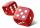What is the probability that two dice throw twice in a row will result the sum of 10?
• Probabilities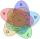If probabilities of A, B and A ∩ B are P (A) = 0.62 P (B) = 0.78 and P (A ∩ B) = 0.26 calculate the following probability (of union. intersect and opposite and its combinations):
• The diceWhat is the probability of events that if we throw a dice is rolled less than 6?
• Three dice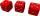What is the probability that when we roll three dice, we roll the numbers 1,2,3?
• Seagull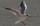Artificially created island in the shape of a circle with a radius of 50 m is overgrown with grass. The only exception is a landing area for helicopters in the shape of a rectangle measuring 15 m and 8 m. What is the probability that the flying seagull (w
• Probability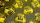What are the chances that the lottery, in which the numbers are drawn 5 of 50 you win the first prize?
• BallsThe urn is 8 white and 6 black balls. We pull 4 randomly balls. What is the probability that among them will be two white?
• Components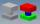In the box are 8 white, 4 blue and 2 red components. What is the probability that we pull one white, one blue and one red component without returning?
• What is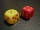What is the probability that the sum of 9 will fall on a roll of two dice? Hint: write down all the pairs that can occur as follows: 11 12 13 14 15. . 21 22 23 24. .. . 31 32. .. . . . . . .. . 66, count them, it's the variable n variable m: 36, 63,. .. .
• Screws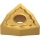The box contains 8 iron, 6 brass, and 4 titanium screws. What is the probability that the randomly selected screw will not be brass?
• Today in schoolThere are 9 girls and 11 boys in the class today. What is the probability that Suzan will go to the board today?